Image Processing Toolbox User's GuideUsing a Gray-Level Co-Occurrence Matrix (GLCM)

The texture filter functions provide a statistical view of texture based on the image histogram. These functions can provide useful information about the texture of an image but cannot provide information about shape, i.e., the spatial relationships of pixels in an image.

Another statistical method that considers the spatial relationship of pixels is the gray-level co-occurrence matrix (GLCM), also known as the gray-level spatial dependence matrix. The toolbox provides functions to create a GLCM and derive statistical measurements from it.

This section includes the following topics.

Creating a Gray-Level Co-Occurrence Matrix

To create a GLCM, use the `graycomatrix` function. The `graycomatrix` function creates a gray-level co-occurrence matrix (GLCM) by calculating how often a pixel with the intensity (gray-level) value i occurs in a specific spatial relationship to a pixel with the value j. By default, the spatial relationship is defined as the pixel of interest and the pixel to its immediate right (horizontally adjacent), but you can specify other spatial relationships between the two pixels. Each element (i,j) in the resultant `glcm` is simply the sum of the number of times that the pixel with value i occurred in the specified spatial relationship to a pixel with value j in the input image.

Because the processing required to calculate a GLCM for the full dynamic range of an image is prohibitive, `graycomatrix` scales the input image. By default, `graycomatrix` uses scaling to reduce the number of intensity values in grayscale image from 256 to eight. The number of gray levels determines the size of the GLCM. To control the number of gray levels in the GLCM and the scaling of intensity values, using the `NumLevels` and the `GrayLimits` parameters of the `graycomatrix` function. See the `graycomatrix` reference page for more information.

The gray-level co-occurrence matrix can reveal certain properties about the spatial distribution of the gray levels in the texture image. For example, if most of the entries in the GLCM are concentrated along the diagonal, the texture is coarse with respect to the specified offset. You can also derive several statistical measures from the GLCM. See Deriving Statistics from a GLCM for more information.

To illustrate, the following figure shows how `graycomatrix` calculates the first three values in a GLCM. In the output GLCM, element (1,1) contains the value `1` because there is only one instance in the input image where two horizontally adjacent pixels have the values `1` and `1`, respectively. `glcm(1,2)` contains the value `2` because there are two instances where two horizontally adjacent pixels have the values `1` and `2`. Element (1,3) in the GLCM has the value 0 because there are no instances of two horizontally adjacent pixels with the values 1 and 3. `graycomatrix` continues processing the input image, scanning the image for other pixel pairs (i,j) and recording the sums in the corresponding elements of the GLCM.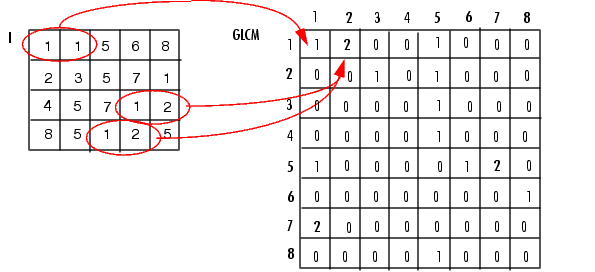Process Used to Create the GLCM

Specifying the Offsets

By default, the `graycomatrix` function creates a single GLCM, with the spatial relationship, or offset, defined as two horizontally adjacent pixels. However, a single GLCM might not be enough to describe the textural features of the input image. For example, a single horizontal offset might not be sensitive to texture with a vertical orientation. For this reason, `graycomatrix` can create multiple GLCMs for a single input image.

To create multiple GLCMs, specify an array of offsets to the `graycomatrix` function. These offsets define pixel relationships of varying direction and distance. For example, you can define an array of offsets that specify four directions (horizontal, vertical, and two diagonals) and four distances. In this case, the input image is represented by 16 GLCMs. When you calculate statistics from these GLCMs, you can take the average.

You specify these offsets as a p-by-2 array of integers. Each row in the array is a two-element vector, `[row_offset, col_offset]`, that specifies one offset. `row_offset` is the number of rows between the pixel of interest and its neighbor. `col_offset` is the number of columns between the pixel of interest and its neighbor. This example creates an offset that specifies four directions and 4 distances for each direction. For more information about specifying offsets, see the `graycomatrix` reference page.

• ```offsets = [ 0 1; 0 2; 0 3; 0 4;...
-1 1; -2 2; -3 3; -4 4;...
-1 0; -2 0; -3 0; -4 0;...
-1 -1; -2 -2; -3 -3; -4 -4];
```
1. The figure illustrates the spatial relationships of pixels that are defined by this array of offsets, where `D` represents the distance from the pixel of interest.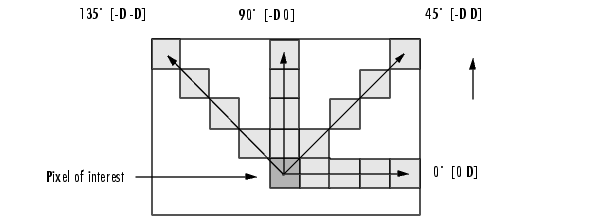Deriving Statistics from a GLCM

After you create the GLCMs, you can derive several statistics from them using the `graycoprops` function. These statistics provide information about the texture of an image. The following table lists the statistics you can derive. You specify the statistics you want when you call the `graycoprops` function. For detailed information about these statistics, see the `graycoprops` reference page.

 Statistic Description Contrast Measures the local variations in the gray-level co-occurrence matrix. Correlation Measures the joint probability occurrence of the specified pixel pairs. Energy Provides the sum of squared elements in the GLCM. Also known as uniformity or the angular second moment. Homogeneity Measures the closeness of the distribution of elements in the GLCM to the GLCM diagonal.

Example: Plotting the Correlation

This example shows how to create a set of GLCMs and derive statistics from them and illustrates how the statistics returned by `graycoprops` have a direct relationship to the original input image.

1. Read in a grayscale image and display it. The example converts the truecolor image to a grayscale image and then rotates it 90° for this example.
• ```circuitBoard = rot90(rgb2gray(imread('board.tif')));
imshow(circuitBoard)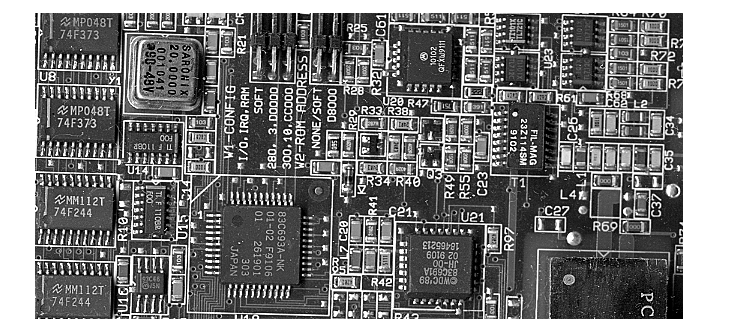```
2. Define offsets of varying direction and distance. Because the image contains objects of a variety of shapes and sizes that are arranged in horizontal and vertical directions, the example specifies a set of horizontal offsets that only vary in distance.
• ```offsets0 = [zeros(40,1) (1:40)'];
```
3. Create the GLCMs. Call the `graycomatrix` function specifying the offsets.
• ```glcms = graycomatrix(circuitBoard,'Offset',offsets0)
```
4. Derive statistics from the GLCMs using the `graycoprops` function. The example calculates the contrast and correlation.
• ```stats = graycoprops(glcms,'Contrast Correlation');
```
5. Plot correlation as a function of offset.
• ```figure, plot([stats.Correlation]);
title('Texture Correlation as a function of offset');
xlabel('Horizontal Offset')
ylabel('Correlation')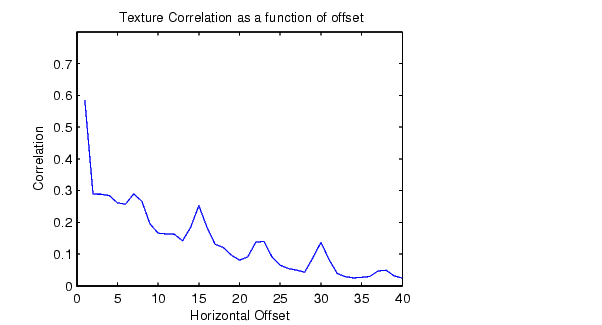The plot contains peaks at offsets 7, 15, 23, and 30. If you examine the input
image closely, you can see that certain vertical elements in the image have
a periodic pattern that repeats every seven pixels. The following figure
shows the upper left corner of the image and points out where this pattern
occurs.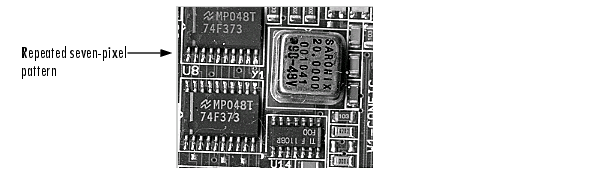```Using Texture Filter Functions Intensity Adjustment